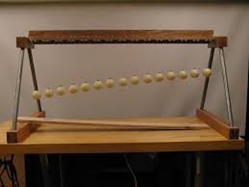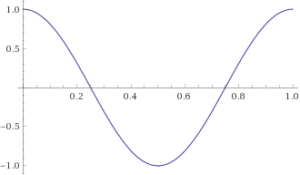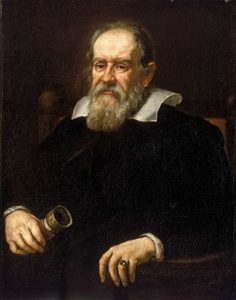# Pendulum WaveRelease the pendulums and observe the beautiful patterns as the pendulum move in-and-out of synch. What kinds of different patterns do you observe? Make a list of what they are and when they occur. In one complete cycle, the first four pendulums have done 31, 32, 33 and 34 swings. How many swings have they completed at the halfway point? How many swings have they completed a quarter of the way through? Can you explain some of the patterns you see?
Maths

The position of a pendulum, during one full swing, looks like this:This is called a cosine curve.

Longer pendulums take longer to complete a cycle, which is why the pendulums gradually move out of synch. However, at various times, parts of their individual cycles start to coincide.

For example, halfway through the dance the pendulums fall into two groups, at opposite amplitudes. Or, a quarter though the dance the pendulums fall into four groups, at different quarter-phases of their cycle. Sometimes the pendulums are at seemingly unrelated points in their cycles and so the pattern looks chaotic.

In 35 seconds, each pendulum has completed a full number of swings (from 31 to 45). At which point all the pendulums are back to where they started and the dance is complete.

History

Although pendulums have been used for thousands of years, Italian scientist Galileo Galilei was the first to study the properties of pendulums around 1602. Galileo discovered that the period of a pendulum does not depend on the weight, but does depend on the square-root of its length, and conceived of the idea for using pendulums for time-keeping. Pendulum clocks were the most accurate method of timekeeping for the next 300 years.
The position of a pendulum follows a cosine curve. Cosine was fully investigated in the 9th century by Arabic scholars. Along with sine and tangent, these are some of the most fundamental functions in mathematics.

PeopleAl-Khwarizmi 780 – 850 Al-Khwarizmi was a Persian astronomer, geologist, and mathematician. He is the inventor of Algebra as a method for solving problems. He produced tables of values for sine, cosine and tangent functions, which describe the motion of pendulums. He was also the first to express fractions as decimals.Galileo Galilei 1564 – 1642 Galileo was an Italian astronomer, physicist, and engineer. Galileo believed the Earth orbits the sun, rather than the other way around. This was considered heretical at the time and Galileo was forced to recant. As a young man Galileo notice how good a pendulum would be for keeping time and began to investigate its properties.
Applications

The mathematics of pendulums can be found in anything with a simple back and forth motion, such as waves going up and down, or when people play on a swing.

Pendulums are often found in clocks, where they were most accurate way to keep time for 300 years. They are also used in scientific devices such as those that measure acceleration or earthquake activity.
The mathematics of the pendulum is the cosine curve. Cosine, sine and tangent are fundamental functions in mathematics and can be found everywhere, from astronomy and physics to engineering and statistics.

Maths at Home

You can make a pendulum at home with some string, and something like plasticine as the weight. It is best if you attach the top of the pendulum to some kind of hook. Investigate how changing the weight, the length of the string, or the angle of release affects the period. You may need a protractor.
Or, investigate how these things affect a pendulum with this online simulation.